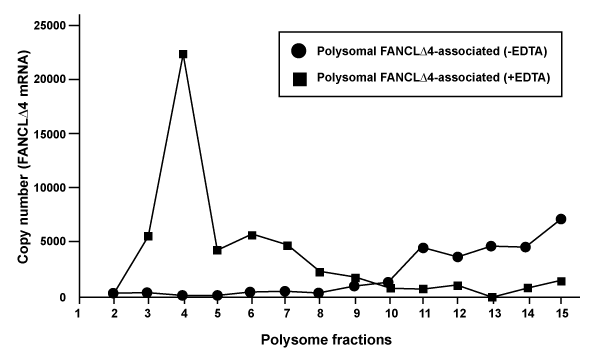Figure 4: Polysome analysis of FANCLΔ4 mRNA. Fractionation of monosomes and polysomes (with or without EDTA) as measured by A260. q-RT PCR of the FANCLΔ4 isoform was performed on T-47D cells and relative expression values for each fraction were calculated by the equation R = (E)(Ctref-Ct), where Ctref is the average cycle threshold of all the fractions for this isoform. The square line is the data acquired from the +EDTA sample whereas the circle line is the data from the -EDTA sample, which illustrates the binding of the FANCLΔ4 transcript with polysomes.